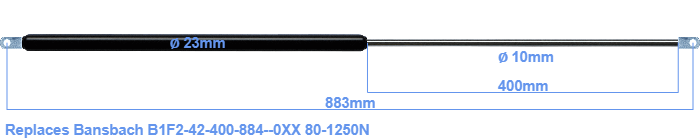# Replacement for Bansbach B1F2-42-400-884–0XX 80-1250N

\$45.49

Replacement gas spring for the Bansbach B1F2-42-400-884--0XX 80-1250 Newton. The eye has a thickness of 10mm and a hole diameter of 8.1mm. Brand: Stabilus Industry Line.

Please note:
• Mounting part rod : The thickness of the eye of this replacement is 10mm instead of 12mm.
• The diameter of the tube of this replacement gas spring is 23mm instead of 22mm.
•  Force Choose an option80 Newton100 Newton120 Newton140 Newton150 Newton160 Newton180 Newton200 Newton220 Newton240 Newton250 Newton260 Newton280 Newton300 Newton320 Newton340 Newton350 Newton360 Newton380 Newton400 Newton420 Newton440 Newton450 Newton460 Newton480 Newton500 Newton520 Newton540 Newton550 Newton560 Newton580 Newton600 Newton620 Newton640 Newton650 Newton660 Newton680 Newton700 Newton720 Newton740 Newton750 Newton760 Newton780 Newton800 Newton820 Newton840 Newton850 Newton860 Newton880 Newton900 Newton920 Newton940 Newton950 Newton960 Newton980 Newton1000 Newton1020 Newton1040 Newton1050 Newton1060 Newton1080 Newton1100 Newton1120 Newton1140 Newton1150 Newton1160 Newton1180 Newton1200 Newton1220 Newton1240 Newton1250 NewtonClearThe diameter of the cylinder of this gas spring is 23 mm. The diameter of the rod is 10 millimeter. The rod (the chrome part) is 400 mm long. In total, the length is 883 millimeter. Note: this is the total length between the rotation points of the two mounting parts. Without the mounting parts this gas spring is 845 mm long (thread to thread). The force of this replacement is 80-1250 Newton. Attention: this is not an official Bansbach gas spring, but a replacement gas spring. This gas spring was produced by Stabilus subsidiary Hahn with the brand Stabilus Industry Line. The force (80-1250 N) and dimensions are close to identical to the original.